# View Multidimensional Signals Using the Array Plot

You can use an Array plot to view multidimensional signals, including variable-size signals, that you log from simulation or import from another source in the Simulation Data Inspector. This example plots and analyzes imported data from a system that:

1. Samples a signal contaminated with noise using an analog to digital converter.

2. Performs a discrete Fourier transform (DFT) on the sampled signal.

3. Removes the noise from the signal in the frequency domain.

4. Performs an inverse DFT on the filtered signal to return to the time domain.

The signal samples are processed using frames, which buffer several samples into an array. You can use the Array plot to view the frames of the time domain signal and to view the frequency domain representation of the signal.

### Import the Data into the Simulation Data Inspector

The data for this example is stored in a MAT file. Import the MAT file into the Simulation Data Inspector using the `Simulink.sdi.Run.create` function or using the Simulation Data Inspector UI. When you import data using the UI, the run is automatically named. You can change the name after importing the data by double-clicking the run name and specifying a new one. When you import data programmatically, you can specify the name for the run.

`runObj = Simulink.sdi.Run.create('Noise Filtering Data Run','file','NoiseFilteringData.mat');`

To import the data using the UI, open the Simulation Data Inspector.

`Simulink.sdi.view;`

In the Simulation Data Inspector, click Import.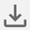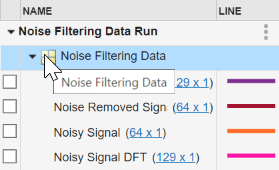In the Import dialog box, select the option to import from a file. Click the folder icon and select the file `NoiseFilteringData.mat`, then click Import.

### Plot the Data Using the Array Plot

By default, the Simulation Data Inspector groups signals according to data hierarchy, and groups of signals are collapsed. To view the signals that were imported, click the arrow next to Noise Filtering Data. Because the signals contain multidimensional data, the dimensions of each sample are indicated to the right of the signal name.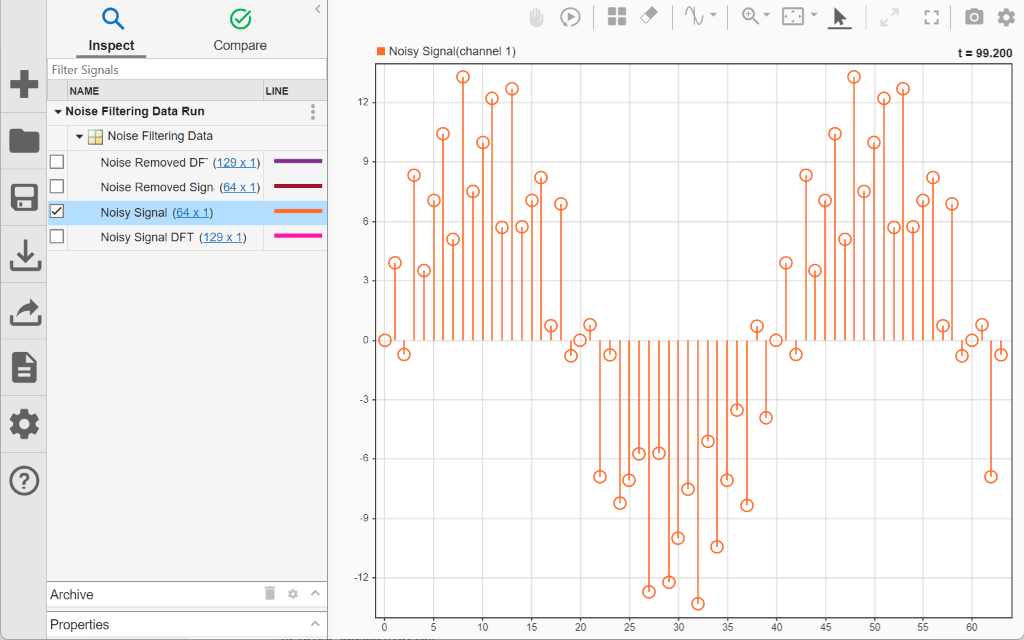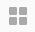To plot `Noisy Signal`, select the check box next to it. A dialog appears in the plot area with actions you can perform on the multidimensional signal. By default, the Simulation Data Inspector uses time plots for all subplots in the layout. Time plots do not support displaying multidimensional data. To view the signal data, select Change active subplot to array plot and click OK.

Each `64`-by-`1` sample of `Noisy Signal` is a frame of time-domain data for a sine wave contaminated by noise. The array plot uses the row index of the sample as the x data and the sample value as the y data.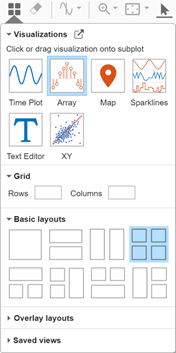To plot the other signals, first change the subplot layout to include four subplots. Click Layout. Then, under Basic Layouts, select the four-subplot layout.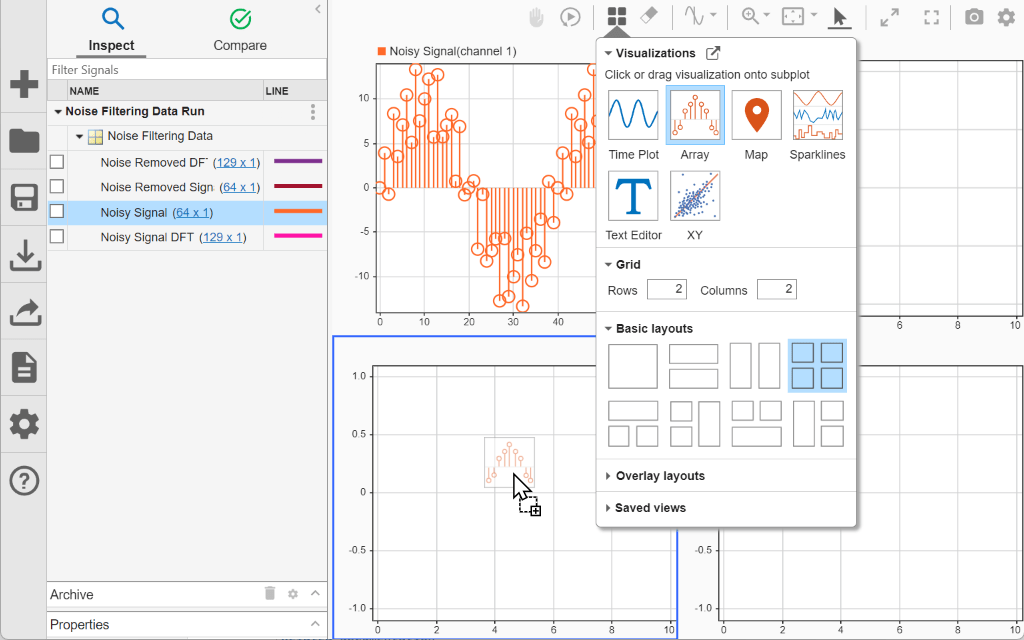You can plot the other signals the same way as the first:

1. Select the subplot where you want to plot the signal.

2. Select the check box next to the signal you want to plot.

3. Select the option to change the active subplot to an array plot.

You can also start by changing the time plots to array plots manually. To manually change a time plot to an array plot, click Layout then select Edit View. From the Visualization Gallery, drag and drop the Array icon onto a subplot.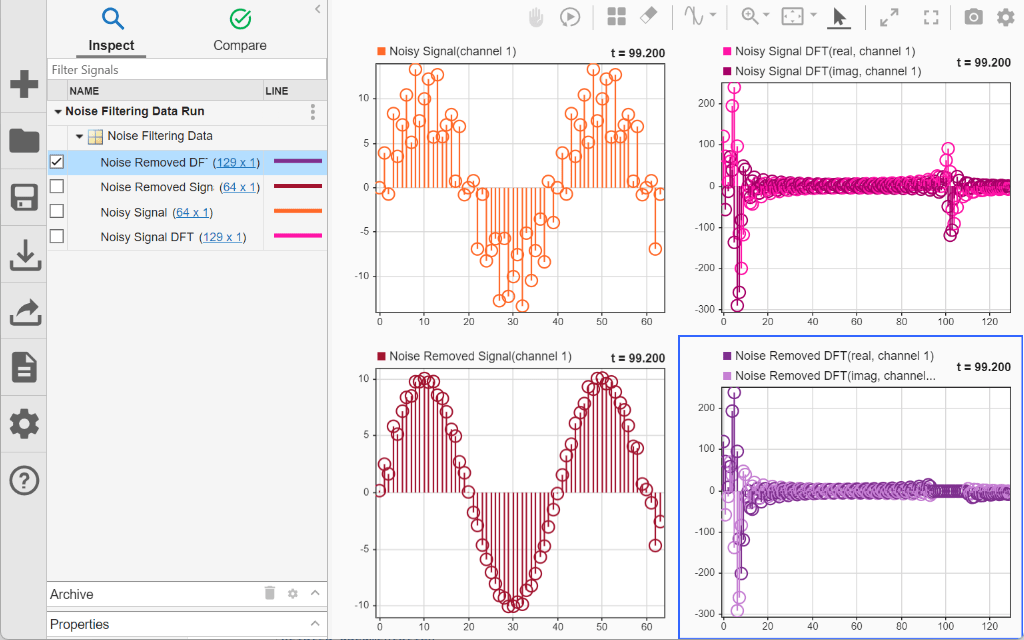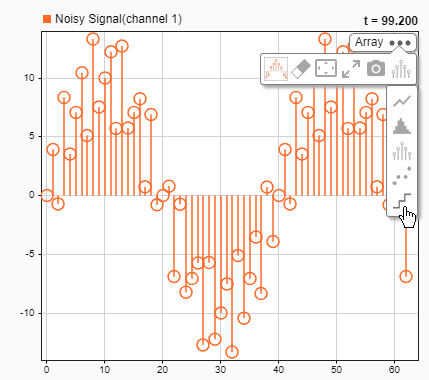Using either strategy, plot the remaining signals with the time-domain signals on the right and then frequency-domain data on the right. The frequency domain data is complex, and the array plot uses different shades of the same color to display the real and imaginary components separately. In the time domain, you can see the sine wave is smoother after the frequency domain processing. In the frequency domain, you can see the peak that corresponds to the high-frequency noise and its removal.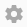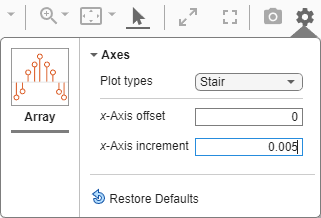### Modify the Array Plot Settings

You can modify how the array plot displays the data by changing the plot type, and you can control the values on the x-axis by specifying an offset and an increment value that defines the space between values on the x-axis. For example, change the settings for the time domain plots to better suit the plotted data.

By default, the array plot uses a stem plot type. Because the frequency domain data is discrete, a stem plot makes sense. The time domain data represents an analog signal that is sampled, which is better represented as a stair plot. You can change the plot type using the subplot menu. To change the `Noisy Signal` array plot type to stair:

1. Pause on the subplot that displays `Noisy Signal`.

2. Click the three dots that appear.

3. Expand the Plot Type list and select Stair.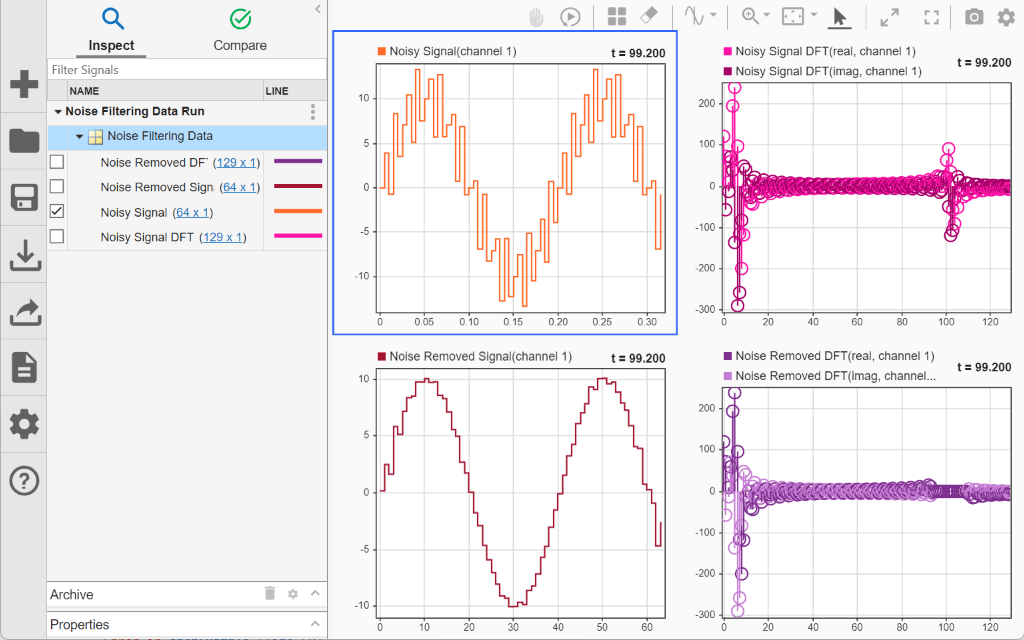Then, change the plot type for the array plot that displays `Noise Removed Signal` to stair as well.

Next, adjust the x-axes for the time domain plots to accurately reflect the relative sample times for each frame. Change the x-axis increment to match the sample rate of `0.005`s used to generate the data using the array plot settings. First, select the subplot you want to modify, then open the Visualization Settings menu and specify the X-Axis Increment.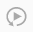The x-axis labels update to indicate that each frame represents a bit more than 300ms of signal data.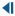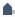### Analyze the Multidimensional Data Over Time

The array plot provides a two-dimensional representation of one sample of a multidimensional signal, plotting the values in a column evenly spaced along the x-axis. The signals also have a time component, and the data in each multidimensional sample can vary with time.

When a view contains visualizations that support cursors, the array plot synchronizes with the cursor to display the sample that corresponds to the cursor position in time. You can also use the replay controls to analyze the sample values on an array plot over time.

The array plot indicates the time that corresponds to the plotted sample in the upper-right of the plot. By default, the array plot displays the last sample of data. When you enable the replay controls, the array plots update to display the sample value for the first time point. To show the replay controls, click Show/hide replay controls.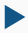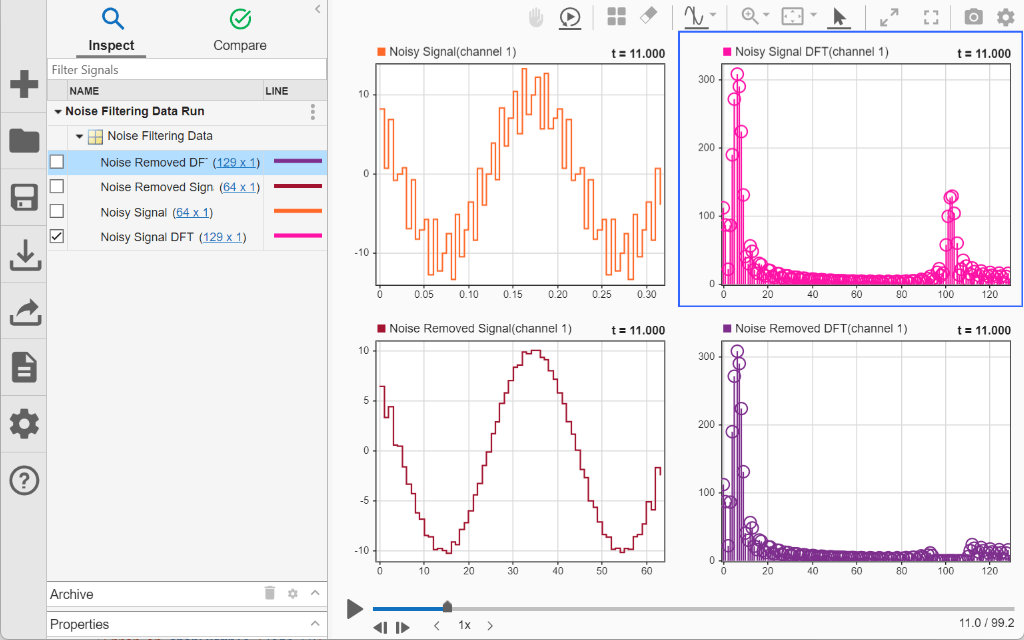You can manually control which sample is displayed on the plots using the Step forward and Step backward buttons or by dragging the progress indicator. To replay the data, press the Replay button.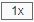The replay sweeps through the sample values at a rate you can adjust using the arrows on either side of the replay speed or specify by clicking the replay speed and entering a new value. By default, the replay updates plots matching one second of time in the data to one second of wall clock time.

As the plots update, you can see how the sine wave shifts within each frame and how that affects the results of the DFT.